# High School Math : Conceptualizing Derivatives

## Example Questions

### Example Question #4 : Techniques Of Antidifferentiation

The speed of a car traveling on the highway is given by the following function of time: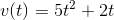Note that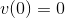What does this mean?

The car is not decelerating at time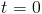.

The car's speed is constantly changing at time.

The car is not accelerating at time.

The car takesseconds to reach its maximum speed.

The car is not moving at time.

The car is not moving at time.

Explanation:

The function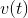gives you the car's speed at time. Therefore, the fact thatmeans that the car's speed isat time. This is equivalent to saying that the car is not moving at time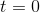. We have to take the derivative of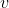to make claims about the acceleration.

### Example Question #11 : Calculus I — Derivatives

The speed of a car traveling on the highway is given by the following function of time: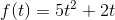Consider a second function: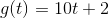It has no relation to the previous function.

It represents another way to write the car's speed.

It represents the rate at which the speed of the car is changing.

It represents the total distance the car has traveled at time.

It represents the change in distance over a given time.

Notice that the function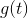is simply the derivative of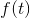with respect to time. To see this, simply use the power rule on each of the two terms.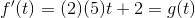Therefore,is the rate at which the car's speed changes, a quantity called acceleration.